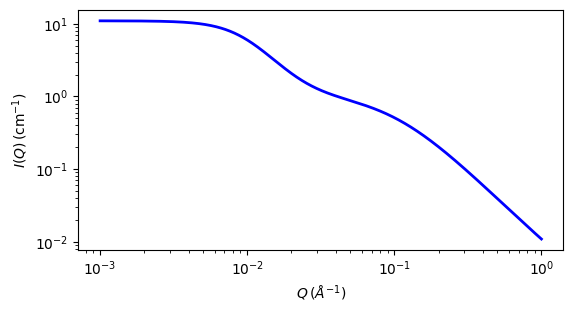# two_lorentzian

This model calculates an empirical functional form for SAS data characterized by two Lorentzian-type functions.

Parameter

Description

Units

Default value

scale

Scale factor or Volume fraction

None

1

background

Source background

cm-1

0.001

lorentz_scale_1

First power law scale factor

None

10

lorentz_length_1

First Lorentzian screening length

100

lorentz_exp_1

First exponent of power law

None

3

lorentz_scale_2

Second scale factor for broad Lorentzian peak

None

1

lorentz_length_2

Second Lorentzian screening length

10

lorentz_exp_2

Second exponent of power law

None

2

The returned value is scaled to units of cm-1 sr-1, absolute scale.

Definition

The scattering intensity $$I(q)$$ is calculated as

$I(q) = \frac{A}{1 +(Q\xi_1)^n} + \frac{C}{1 +(Q\xi_2)^m} + \text{B}$

where $$A$$ = Lorentzian scale factor #1, $$C$$ = Lorentzian scale #2, $$\xi_1$$ and $$\xi_2$$ are the corresponding correlation lengths, and $$n$$ and $$m$$ are the respective power law exponents (set $$n = m = 2$$ for Ornstein-Zernicke behaviour).

For 2D data the scattering intensity is calculated in the same way as 1D, where the $$q$$ vector is defined as

$q = \sqrt{q_x^2 + q_y^2}$Fig. 123 1D plot corresponding to the default parameters of the model.

Source

two_lorentzian.py

References

None.

Authorship and Verification

• Author: NIST IGOR/DANSE Date: pre 2010